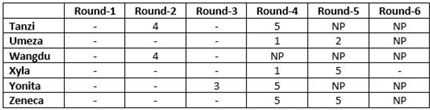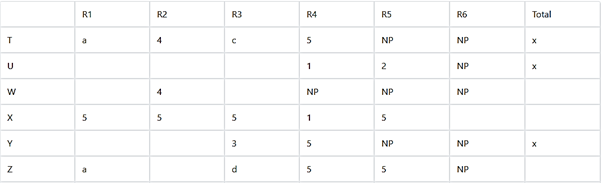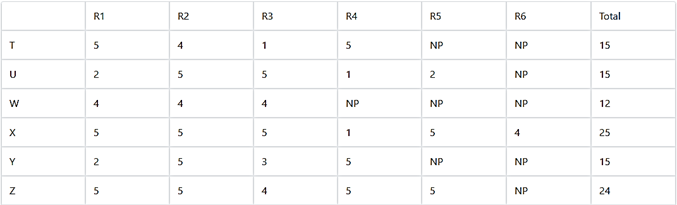### CAT 2019 - Slot 1 - Logical Reasoning and Data Interpretation - Six players – Tanzi, Umeza, Wangdu, Xyla, Yonita and Zeneca competed in an archery tournament

\$ 39
99
Monthly
• List Item #1
• List Item #2
Popular
##### Six players – Tanzi, Umeza, Wangdu, Xyla, Yonita and Zeneca competed in an archery tournament. The tournament had three compulsory rounds, Rounds 1 to 3. In each round every player shot an arrow at a target. Hitting the centre of the target (called bull’s eye) fetched the highest score of 5. The only other possible scores that a player could achieve were 4, 3, 2 and 1. Every bull’s eye score in the first three rounds gave a player one additional chance to shoot in the bonus rounds, Rounds 4 to 6. The possible scores in Rounds 4 to 6 were identical to the first three. A player’s total score in the tournament was the sum of his/her scores in all rounds played by him/her. The table below presents partial information on points scored by the players after completion of the tournament. In the table, NP means that the player did not participate in that round, while a hyphen means that the player participated in that round and the score information is missing.The following facts are also known.1.     Tanzi, Umeza and Yonita had the same total score. 2.     Total scores for all players, except one, were in multiples of three. 3.     The highest total score was one more than double of the lowest total score. 4.     The number of players hitting bull’s eye in Round 2 was double of that in Round 3. 5.     Tanzi and Zeneca had the same score in Round 1 but different scores in Round 3.

Q. 1: What was the highest total score?
1. 25
2. 23
3. 24
4. 21

##### Q. 2: What was Zeneca’s total score?1. 212. 243. 224. 23

Q. 3: Which of the following statements is true?
1. Zeneca’s score was 23.
2. Xyla was the highest scorer.
3. Xyla’s score was 23.
4. Zeneca was the highest scorer

Q. 4: What was Tanzi’s score in Round 3?
1. 3
2. 4
3. 1
4. 5

1. Option: 1
2. Option: 2
3. Option: 2
4. Option: 1

Explanation:
As from the table given we can see that Xyla had played all the six rounds in means he had scored 5 points in each of first 3 rounds.
Similarly Tanzi and Yonita each had hit one bull’s eye score in first three rounds, Umeza and Zeneca had two bull’s eye score in first 3 rounds while Wangdu didn’t had any bull’s eye score.
So this information can be tabulated as below: (Name of players have been represented by first letter of their name )As number of player hitting bull’s eye in round 2 is double that of the number in round 3. And 2 players have already scored 4 points in Round 2 so maximum possible people hitting bulls eye in Round 3 can be 2 and in round 2 can be 4.

Thus a = 5 as c and d can not be 5.

Possible score of U = 5+5+1+2 + b = 13+b as it should be multiple of 3 so b = 2 thus x = 15

Thus Tanzi’s score in round 3 , c = 15 – 5 – 4 – a = 15 – 5 -4-5 = 1

Also W must have minimum score as his maximum possible score = 12 (if he scores 4 in each of first 3 rounds)Let it be m and X must have maximum possible score let it be n.

As m <= 12 and n >= 22

As n= 2m + 1

S n can be 23 or 25 .

But if n = 23 , m = 11 (which is not possible as only one of the all total score is not multiple of 3)

Thus n = 25 and m = 12

As Z has four scores of 5 so his 5th score will be either 1 or 4. But it can not be 1 as T’s score was 1.

Now the complete table will look like,Now all the question can be answered.

Inspiring Education… Assuring Success!!

Ghatkopar | Borivali | Andheri | Online

## Ⓒ 2016 - All Rights Are Reserved

### CAT 2019 - Slot 1 - Logical Reasoning and Data Interpretation - Six players – Tanzi, Umeza, Wangdu, Xyla, Yonita and Zeneca competed in an archery tournament

\$ 39
99
Monthly
• List Item #1
• List Item #2
Popular
##### Six players – Tanzi, Umeza, Wangdu, Xyla, Yonita and Zeneca competed in an archery tournament. The tournament had three compulsory rounds, Rounds 1 to 3. In each round every player shot an arrow at a target. Hitting the centre of the target (called bull’s eye) fetched the highest score of 5. The only other possible scores that a player could achieve were 4, 3, 2 and 1. Every bull’s eye score in the first three rounds gave a player one additional chance to shoot in the bonus rounds, Rounds 4 to 6. The possible scores in Rounds 4 to 6 were identical to the first three. A player’s total score in the tournament was the sum of his/her scores in all rounds played by him/her. The table below presents partial information on points scored by the players after completion of the tournament. In the table, NP means that the player did not participate in that round, while a hyphen means that the player participated in that round and the score information is missing.The following facts are also known.1.     Tanzi, Umeza and Yonita had the same total score. 2.     Total scores for all players, except one, were in multiples of three. 3.     The highest total score was one more than double of the lowest total score. 4.     The number of players hitting bull’s eye in Round 2 was double of that in Round 3. 5.     Tanzi and Zeneca had the same score in Round 1 but different scores in Round 3.

Q. 1: What was the highest total score?
1. 25
2. 23
3. 24
4. 21

##### Q. 2: What was Zeneca’s total score?1. 212. 243. 224. 23

Q. 3: Which of the following statements is true?
1. Zeneca’s score was 23.
2. Xyla was the highest scorer.
3. Xyla’s score was 23.
4. Zeneca was the highest scorer

Q. 4: What was Tanzi’s score in Round 3?
1. 3
2. 4
3. 1
4. 5

1. Option: 1
2. Option: 2
3. Option: 2
4. Option: 1

Explanation:
As from the table given we can see that Xyla had played all the six rounds in means he had scored 5 points in each of first 3 rounds.
Similarly Tanzi and Yonita each had hit one bull’s eye score in first three rounds, Umeza and Zeneca had two bull’s eye score in first 3 rounds while Wangdu didn’t had any bull’s eye score.
So this information can be tabulated as below: (Name of players have been represented by first letter of their name )As number of player hitting bull’s eye in round 2 is double that of the number in round 3. And 2 players have already scored 4 points in Round 2 so maximum possible people hitting bulls eye in Round 3 can be 2 and in round 2 can be 4.

Thus a = 5 as c and d can not be 5.

Possible score of U = 5+5+1+2 + b = 13+b as it should be multiple of 3 so b = 2 thus x = 15

Thus Tanzi’s score in round 3 , c = 15 – 5 – 4 – a = 15 – 5 -4-5 = 1

Also W must have minimum score as his maximum possible score = 12 (if he scores 4 in each of first 3 rounds)Let it be m and X must have maximum possible score let it be n.

As m <= 12 and n >= 22

As n= 2m + 1

S n can be 23 or 25 .

But if n = 23 , m = 11 (which is not possible as only one of the all total score is not multiple of 3)

Thus n = 25 and m = 12

As Z has four scores of 5 so his 5th score will be either 1 or 4. But it can not be 1 as T’s score was 1.

Now the complete table will look like,Now all the question can be answered.

Inspiring Education… Assuring Success!!

Ghatkopar | Borivali | Andheri | Online